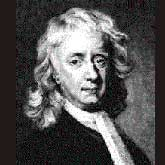# Newton's Three Laws of MotionThe motion of an aircraft through the air can be explained and described by physical principals discovered over 300 years ago by Sir Isaac Newton. Newton worked in many areas of mathematics and physics. He developed the theories of gravitation in 1666, when he was only 23 years old. Some twenty years later, in 1686, he presented his three laws of motion in the 'Principia Mathematica Philosophiae Naturalis.' Newton's 1st law states that every object will remain at rest or in uniform motion in a straight line unless compelled to change its state by the action of an external force. This is normally taken as the definition of inertia. The key point here is that if there is no net force acting on an object (if all the external forces cancel each other out) then the object will maintain a constant velocity. If that velocity is zero, then the object remains at rest. If an external force is applied, the velocity will change because of the force.

The 2nd law explains how the velocity will change. The law defines a force to be equal to change in momentum (mass times velocity) per change in time. Newton also developed the calculus of mathematics, and the 'changes' expressed in the second law are accurately defined in differential forms. (Calculus can also be used to determine the velocity and location variations experienced by an object subjected to an external force.) For an object with a constant mass, the 2nd law can be more easily expressed as the product of an object's mass and its acceleration (F = ma). For an external applied force, the change in velocity depends on the mass of the object. A force will cause a change in velocity; and likewise, a change in velocity will generate a force. The equation works both ways.

The 3rd law states that for every action (force) in nature there is an equal and opposite reaction. In other words, if object A exerts a force on object B, then object B also exerts an equal force on object A. Notice that the forces are exerted on different objects. The third law can be used to explain the generation of lift by a wing and the production of thrust by a jet engine.

NASA Glenn Research CenterThe NASA Glenn Research Center in Cleveland, Ohio designs and develops innovative technology to advance NASA’s missions in aeronautics and space exploration.

### Science Articles: